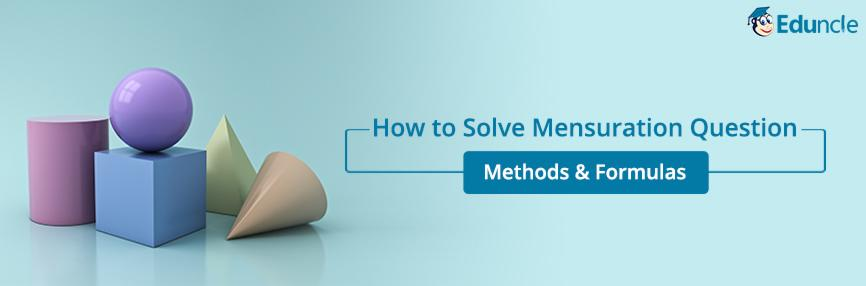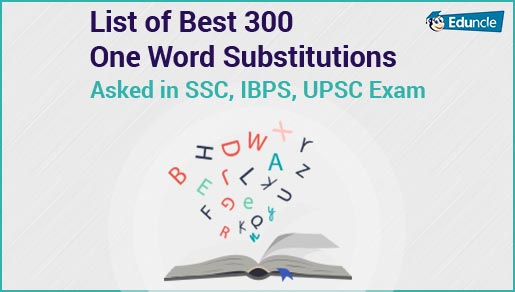Government Jobs FollowRavi @Eduncle posted an Article
June 22, 2019 • 10:19 am

# How to Solve Mensuration Questions – Important Methods & FormulasMensuration is counted in the list of important topics of Aptitude section for all type of competitive exams.

This topic is completely based on the calculations and formulas.

Aspirants who are preparing for exams like SSC, Railway and Bank, should focus on this topic as the questions are likely to come from this.

Here in this blog, we are going to discuss some important Mensuration Formulas which are used to solve the complicated Mensuration Problems.

Read the complete blog to get the 100% benefit.

Start Preparation of Bank Exams 2019 with Free Study Stuff - Download Now!

## What is Mensuration in Math?

As per the definition ‘Measuring the area of surface, length of lines and volumes with the provided data is counted in Mensuration’.

Mensuration is used to measure the important physical quantities of geometrical figures.

It deals with the area, length and volume of shapes like 2d and 3D.

We hope that you are now well aware of the term ‘Mensuration’. For in-depth knowledge of this topic, you need to scroll down the blog.

## List of Basic Conversions to Solve Mensuration Questions

Candidates will have to convert some units as per the requirements in the questions.

In most of the questions of Mensuration, you will require to change the Units of given values to get the desired answer.

Below we have shared some important conversions that are used to solve the mensuration problems.

 Sector Conversions Area 1 Hactare = 10000 m2 1 Decametre = 100 m2 1 Centimeter square = 1/10000 m2 1 kilometre square = 1000000 m2 1 Milimeter square = 1/000000 m2 1 Decimeter square = 1/100 m2 Volume 1 litre = 1000 cm³ 1 Hectometer³ = 10000 m³ 1 Milimeter³ = 1/100 cm³ 1 Decameter³ = 100 m³ 1 Meter³ = 1000000 cm³

It doesn’t matter for which exam you are preparing for, the knowledge of the above-mentioned conversions is essential for solving the quantitative questions.

As now you are well aware of the important conversions used to solve the mensuration questions, it’s time to learn some important Mensuration Formulas.

Below we have provided a complete list of formulas that all can be used to attempt different types of Mensuration questions.

Targeting Upcoming SSC Exams 2019? Get Free Study Materials and Previous Year Question Papers. Download Now.

## List of Mensuration Formulas – Know How to Solve A Difficult Problem Easily!

Mensuration Questions in competitive exams are likely to be asked from some certain areas like Triangle, parallelogram, square circle etc.

Formulas of all these sectors are summed up in a common table given below.

Learn these formulas and start preparing for all the government jobs exams.

 Square Area = a×a Perimeter = 4a Rectangle Area = lb Perimeter = 2(l+b) Triangle Area =bxh/2 or √s(s-a)(s-b)(s-c)…………….where s=a+b+c/2 Circle Area = πr2 or πd2 /4 Circumference = 2πr or πdArea of sector of a circle = (θπr2)/360 Right Angle Triangle Area =1/2(bh) Perimeter = b+h+d Cube Volume: V = l3 Lateral surface area = 4a2 Surface Area: S = 6s2 Diagonal (d) = √3l Sphere Volume: V = (4/3)πr3 Surface Area: S = 4πr2 Hemisphere Volume =(2/3)π r3 Curved surface area (CSA) = 2 π r2 Total surface area = TSA = 3 π r2 Right Circular Cylinder Volume = πr²h Lateral surface Area = 2πrh Whole surface area = (2πrh + 2πr²)

Besides of the formulae that we provided in this table, there are some specific formulas for specific conditions.

Below you can check the formulas to ease out your preparation for government jobs exams.

2h(l+b) will be used to find the area of four walls

2(l+b+4w) will be used to find the Perimeter of Pathway around a rectangle field

2w(l+b-2w) is used to find the area of Pathway inside a rectangle field

w(l+b-w) can be used to get the area of Pathway running across the middle of a rectangle

2(l+b-4w) this formula is used for getting the Perimeter of Pathway inside a rectangle field

2w(l+b+2w) will be used to find the area of Pathway around a rectangle field

For a better explanation of Mensuration Question, below we have provided an example related to the sphere.

Check this out –

Question –

A solid cone of height 9 cm with a diameter of its base 18 cm is cut out from a wooden solid sphere of radius 9 cm. The percentage of wood wasted is.

Solution –

As we know the Volume of Sphere is counted by the formula -  (4/3)πr3

Putting the given values in the formula we get – 4π/3 × 9 × 9 × 9 = 972 π cm3

The volume of Cone -πr2h

Putting the values in the above formula we get -(1/3)π × 9× 9× 9 = 243 π cm3

To find the percentage of wood wasted will be out by deducting the volume of sphere from volume of cone.

So, the Percentage of wood wasted will be -{(972π - 243π)/972π} × 100 = 75%

To solve the Mensuration Problems, all you need is to learn the formulas and a lot of practice.

You can use the sticky notes in your room to learn these formulas.

Use mock test papers for practice. At least 3-4 test series should be attempted by an aspirant in a day for best results.

Start Preparation of Bank Exams 2019 with Free Study Stuff & Video Lectures - Download Now!

Here we have reached at the end of this blog. We have covered almost all important formulas that are sufficient to solve any kind of mensuration problems. We hope you enjoyed learning from this blog.

How was your experience with this blog?

Is it helpful to learn the Mensuration?

You can share your thoughts with us with the help of comments’ box below.

You Might Also Like